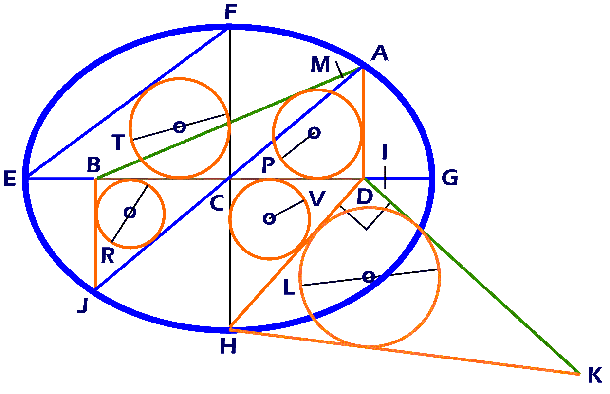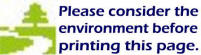Home Page The Unusual Odyssey of the Elliptical Constant
 The LegendLegend of the Lemma Ellipse   Line AB = DK = hypotenuse = h Line AD = BJ = radius = r Line AJ = diagonal = D Line BC = CD = soliton = s Line BD = wave = w Line BG = ED = apogee = o Line CA = CJ = diagonal radial = d Line CF = CH = amplitude = a Line CG = EC major radial = m Line DG = EB = perigee = p Line DH = vector = v Line EF = diameter chord = c Line EG = major diameter = M Line FH = minor diameter = A Line HK = lemma = l \ Line oP = Hypotenuse radius = Hr = Natural integer Line oV = Vector radius = Vr = Natural integer Line oR = Diagonal diameter = Dd = Natural integer Line oT = Chord diameter = Cd = Natural integer Line oL = Lemma diameter = Ld = Natural integer Triangle ABD = Hypotenuse triangle                           = Brunardot triangle Triangle CDH = Vector triangle                           = Pythagorean triangle Triangle BCJ = Diagonal triangle                          = Pythagorean triangle Triangle CEF = Chord triangle                          = Pythagorean triangle Triangle DHK = Lemma triangle                           = Pythagorean triangle Inscribed circle ABD = Hypotenuse circle Inscribed circle CDH = Vector circle Inscribed circle CEF = Chord circle Inscribed circle DHK = Lemma circle p,  s,  v,  o = 1st four terms of a Brunardot Series All lines shown in the above image are integers derived from simple algebraic relationships for an unending sequence of Lemma Ellipses that are generated from two unending sequences of Natural Integers.
 Page Return
 EllipticalConstant.com website The Elliptical Constant (EC) is the Primary, Universal, True Constant that establishes the value of . . ."ONE!" © Copyright 2005-17 by CONCEPTUAL Applications Terms:  Brunardot, and Pulsoid Theory must be cited.CONCEPTUAL Applications Family of Informational Websites  Emphasizing Philogic, a discipline that overarches Science, Theology, and Philosophy (STP) Website Design by:   CONCEPTUAL  Applications a division of: WebComm21